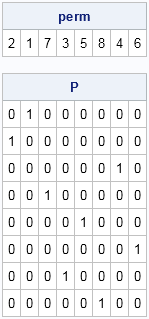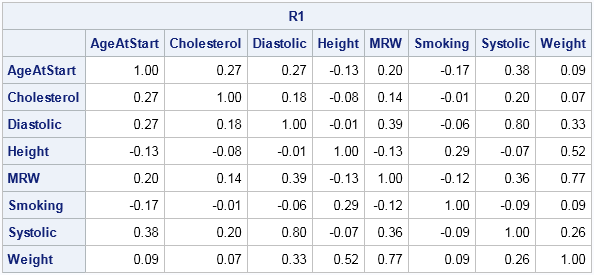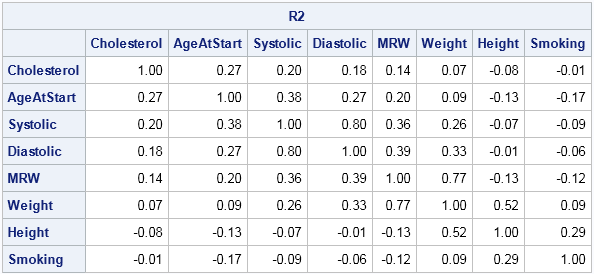Suppose that you compute the correlation matrix (call it R1) for a set of variables x1, x2, ..., x8. For some reason, you later want to compute the correlation matrix for the variables in a different order, maybe x2, x1, x7,..., x6. Do you need to go back to the data and compute the new correlation matrix (call it R2)? Or can you somehow obtain the new correlation matrix from the old one?

The answer, of course, is that you can create the new correlation matrix from the original matrix. After all, R1 contains all of the correlations between variables. The challenge is to figure out how to rearrange the numbers in R1 so that they form R2.

### Permutation matrices

Because the SAS/IML language has built-in support for matrix operations, it is a good tool for converting R1 into R2. Mathematically speaking, this is a classic situation in which a permutation matrix is useful. A permutation matrix is formed by permuting the rows of the identity matrix. I have written about using permutation matrices to create "Secret Santa" lists, where each person in a group gives a present to someone else in the group.

One of the nice properties of a permutation matrix is the ease with which you can permute rows and columns in a second matrix. If P is a permutation matrix and A is any square matrix, then the matrix product P*A permutes the rows of A. Similarly, the multiplication A*P` permutes the columns of A. The transpose of a permutation matrix is also a permutation matrix.

The following SAS/IML module shows one way to generate a permutation matrix when the permutation is represented by a row vector:

```proc iml; /* create permutation matrix from a permutation, v, where v is a row vector */ start PermutationMatrix(v); return( I(ncol(v))[v, ] ); /* generate identity, then reorder rows */ finish;   perm = {2 1 7 3 5 8 4 6}; /* permutation of 1:8 */ P = PermutationMatrix(perm); print perm, P;```The permutation matrix is a reordering of the rows of the identity matrix:

• The first row of P is the second row of the identity matrix because perm=2.
• The second row of P is the first row of the identity matrix because perm=1.
• The third row of P is the seventh row of the identity matrix because perm=7, and so forth.

### Reordering a correlation matrix

Let's see how the permutation matrix enables you to reorder a correlation matrix. The following statements compute a correlation matrix for eight variables in the Sashelp.Heart data set, which records measurements about patients in the Framingham Heart Study. The variables are listed in alphabetical order:

```/* list variables in alphabetical order */ varNames = {"AgeAtStart" "Cholesterol" "Diastolic" "Height" "MRW" "Smoking" "Systolic" "Weight"}; use Sashelp.Heart; read all var varNames into X; close;   R1 = corr(X); print R1[c=varNames r=varNames format=5.2];```The correlation matrix shows the correlations between the alphabetical list of variables. Applying a permutation such as perm will rearrange the variables into a different order. In the same way, you can apply the permutation matrix, P, to permute the rows and columns of R1. In turns out that the correct formula to use is R2 = P*R1*P', as shown below:

```Names = varNames[perm]; R2 = P*R1*P`; print R2[c=Names r=Names format=5.2];```You can verify that R2 is the correct correlation matrix for the reordered variables by repeating the correlation computation: corr(X[,perm]).

Why is this P*R1*P' the correct formula? Well, a permutation matrix is an example of an orthogonal matrix, so P` = P-1. That means that the formula specifies a similarity transformation between R1 and R2 that correspond to a change of bases. The new basis vectors are the specified permutation of the standard basis vectors.

Although I have demonstrated this technique for a correlation matrix, it applies to other matrices such as a covariance matrix or a distance matrix. It can be a useful technique when you rearrange the variable order so that similar variables are clustered together.

### An alternative solution

As demonstrated, you can use premultiplication by a permutation matrix to permute rows, and postmultiplication to permute columns. If you aren't comfortable with permutation matrices, there is an easy alternative way to create R2 by permuting the column and rows subscripts of R1:

```B = R1[,perm]; /* permute the columns */ R2 = B[perm,]; /* permute the rows */ C = R1[perm,perm]; /* permute the rows and the columns */```

So whether you use the permutation matrix to permute the columns and rows of a matrix or whether you permute them directly by using subscripts, the SAS/IML language gives you an easy way to manipulate an array of new multivariate statistics to accommodate a change in the ordering of a list of variables.

Share